# 1st PUC Maths Question Bank Chapter 12 Introduction to Three Dimensional Geometry

Students can Download Maths Chapter 12 Introduction to Three Dimensional Geometry Questions and Answers, Notes Pdf, 1st PUC Maths Question Bank with Answers helps you to revise the complete Karnataka State Board Syllabus and score more marks in your examinations.

## Karnataka 1st PUC Maths Question Bank Chapter 12 Introduction to Three Dimensional Geometry

Question 1.
Define coordinate planes and rectangular coordinate system in space (or 3-D space).
Let X’OX, Y’OY and Z’OZ be three mutually perpendicular lines having the same origin ‘O’. These lines are called X-axis, Y-axis and Z-axis respectively. The plane containing X’OX and Y’OY is called XOY plane (or XY-plane). i.e., the X-axis and Y-axis determine a plane, called XY-plane. the plane containing YOY’ and Z’OZ is called YOZ plane (or YZ-plane) and the plane containing X’OX and Z’OZ is called ZOX plane (or ZX – plane). These planes are called coordinate planes. This system of coordinate axes is called a rectangular coordinate system in space.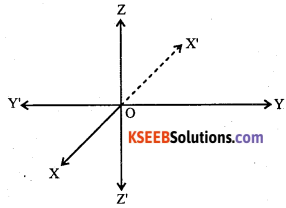Definition: Coordinate planes:
The three planes determined by the pair of axes are called (coordinate planes)

Note:

(i) The coordinates of a point P in 3-D is always written in the form of triplet like
(x, y, z), where x, y and z are the distances from the YZ, ZX and XY planes.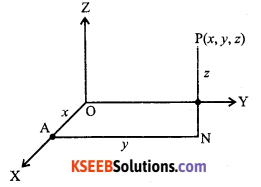(ii) Coordinates of the origin O = (0, 0, 0)
(iii) Any point on the x-axis is of the form (x, 0, 0).
(iv) Any point on the y-axis is of the form (0, y, 0)
(v) Any point on the z-axis is of the form (0, 0, z)
(vi) Equations of x-axis are y = 0 and z = 0
(vii) Equations of y-axis are x = 0 and z = 0 (viii) Equations of z-axis are x = 0 and y = 0
(viii) Equation of XY-plane is z = 0 and any point in XY – plane will be (x, y, 0)
(ix) Equation of YZ – plane is x = 0 and any point in YZ – plane will be (0, y, z).
(x) Equation of ZX – plane is y = 0 and any point in the ZX – plane is (x, 0, z).Question 2.
Define octants.
The three coordinate planes divide the space into eight parts known as octants.

Question 3.
Write the table which shows the signs of the three coordinates in all the eight octants.

 Octant coordinate I II III IV V VI VII VII X + – – + + – – + y + + – – + + – – z + + + + – – – –

Question 4.
Show that the distance between the points (x1, y1, z1) and (x2, y2, z2) is given by
$$\sqrt{\left(x_{2}-x_{1}\right)^{2}+\left(y_{2}-y_{1}\right)^{2}+\left(z_{2}-z_{1}\right)^{2}}$$
Let P(x1,y1,z1) and Q(x2,y2,z2) be the given points.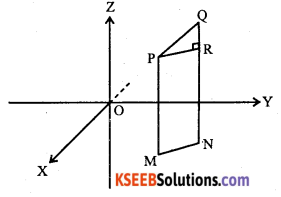Let PQ be the line not parallel to z-axis. Draw PM and QN perpendiculars to XY-plane. Since XY-plane is two dimensional (2 – D), then the coordinates of M and N are respectively (x1 , y1) and (x2 , y2).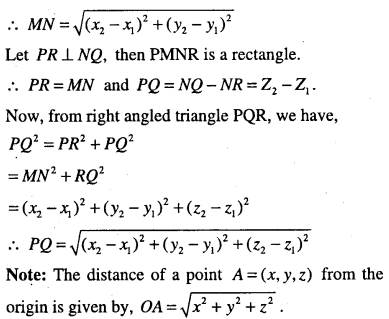Section formula :

Question 5.
If A(x1,y1,zl) and B(x2,y2,z2) are two distinct points in space and a point P(x,y,z) divides AB in the ratio m : n internally then Prove that.
$$x=\frac{m x_{2}+n x_{1}}{m+n}, \quad y=\frac{m y_{2}+n y_{1}}{m+n}, \quad z=\frac{m z_{2}+n z_{1}}{m+n}$$
$$\text { i.e., } P=\left(\frac{m x_{2}+n x_{1}}{m+n}, \frac{m y_{2}+n y_{1}}{m+n}, \frac{m z_{2}+n z_{1}}{m+n}\right)$$
Draw AL, PN and BM perpendiculars to the xy-plane. Clearly the feet of the perpendiculars L, M, N are collinear and L = (x1,y1), M -(x2,y2), N = (x,y). Now, draw a line through P which is parallel to the line LM meets AL produced at C and BM at D. Clearly the triangle PAC and PDB are equiangular and hence similar.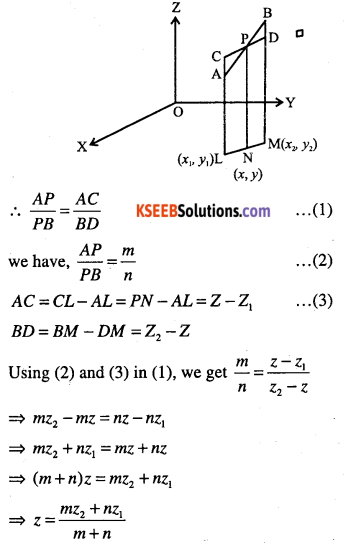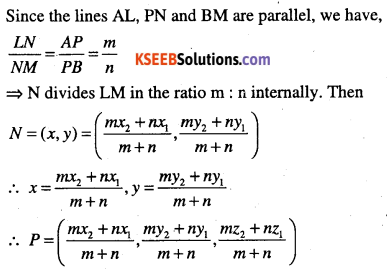Remark:
(i) It P divides AB externally in the ratio m : n, then the coordinates of the point P are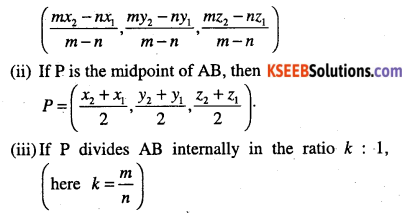Observations:

• The point P divides AB internally in the ratio k : 1 iff k > 0 (i.e., k is + ve)
• The point P divides AB externally in the ratio |k| : 1 iff k < 0 (i.e., k is – ve)Question 6.
Find the co-ordinates of the centroid of the triangle whose vertices are (x1,y1,z1) (x2,y2,z2) and (x3,y3,z3)
Note: Centroid is the point of intersection of the medians of the triangle and it is denoted by G. Centroid divides the median in the ratio 2:1.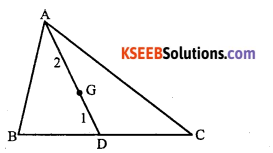Let A = (x1,y1,z1) , B = (x2,y2,z2) and C = (x3, y3, z3) be the vertices of the triangle ABC. Let AD be the median drawn from A. Then D is the mid-point of BC.
∴ D=$$\left(\frac{x_{2}+x_{3}}{2}, \frac{y_{2}+y_{3}}{2}, \frac{z_{2}+z_{3}}{2}\right)$$
Let G be the centroid of the ΔABC. median AD in the ratio 2 : 1.
∴ The coordinates of G are,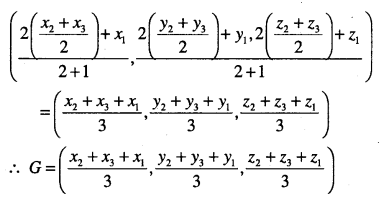Question 7.
Find the ratio in which the
(i) xy – plane
(ii) yz – plane
(iii) zx – plane divides the line segment formed by joining the points (x1,y1,z1) and (x2,y2,z2).
We have, the coordinates of a point dividing the line joining the points A (x1,y1,z1) and
B (x2,y2,z2) in the ratio k : 1 are,
$$P=\left(\frac{k x_{2}+x_{1}}{k+1}, \frac{k y_{2}+y_{1}}{k+1}, \frac{k z_{2}+z_{1}}{k+1}\right)$$

(i) If xy – plane divides a line segment joining the given points, A, B, then z – coordinate of P is zero.
$$\text { i.e., } \frac{k z_{2}+z_{1}}{k+1}=0 \Rightarrow k z_{2}+z_{1}=0 \Rightarrow k=\frac{-z_{1}}{z_{2}}$$
∴ xy-plane divides the line segment AB in the ratio -z1 : z2
Similarly,
(ii) The yz-plane in the ratio -x1: x2
(iii) The zx-plane in the ratio -y1 : y2Question 8.
A point is on the x-axis. What are its y and z – coordinates?
Since a point is on the x-axis then it is of the form (x,0,0)
∴  y-coordinate = 0 and z-coordinate = 0.

Question 9.
A point is in the xz-plane. What can you say about its y-coordinate?
Since a point is in the xz-plane, then it is of the form (x,0, z). So its y-coordinate is zero.

Question 10.
Name the octants in which the following points he. (1, 2, 3), (2, 4, 5), (-3, 1, 2), (-3, 1, -2), (4, -2, 3), (4, -2, -5), (4, 2, -5), (-4, 2, -5), (-4,2, 5), (-3,-1,6), (-2,-4,-7).

 Sl.No Point Octant (i) (1,2, 3) first octant (ii) (2, 4, 5) first octant (iii) (-3,1,2) second octant (iv) (-3,1,-2) sixth octant (v) (4,-2, 3) fourth octant (vi) (4,-2,-5) eighth octant (vii) (4, 2,-5) fifth octant (viii) (-4, 2, -5) sixth octant (ix) (-4, 2, 5) second octant (x) (-3, -1,6) third octant (xi) (-2, -4, -7) seventh octant

Question 11.
Find the distance between the following pairs of points.
(i) (1,-3,4) and (-4,1,2)
(ii) (2, 3, 5) and (4,3,1)
(iii) (-3,7,2) and (2, 4,-1)
(iv) (-1,3, -4) and (1,-3,4)
(v) (2,-1,3) and (-2,1,3)
(i) Let A (1, -3, 4) and B = (-4, 1, 2) be the given points.
Distance between the points A and B is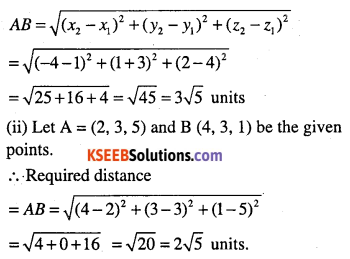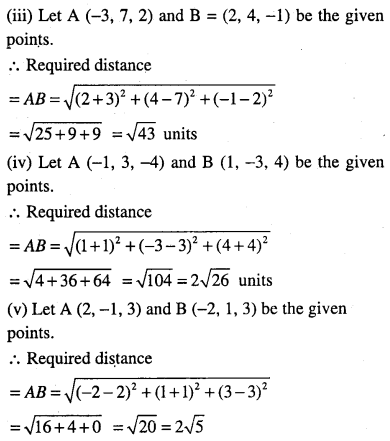Question 12.
Show that the following points are collinear.
(i) (-2,3,5), (1,2,3) and (7, 0,-1)
(ii) (1,2,4), (3, 8,8), (7,20,16)
(i) Let A (-2, 3, 5), B (1, 2, 3) and C (7, 0, -1) be the given points. We know that points are said to be collinear if they lie on a line.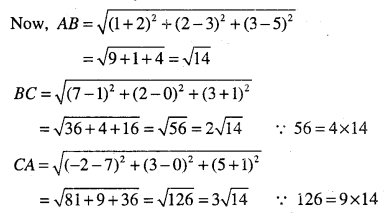$$\text { Thus } A B+B C=\sqrt{14}+2 \sqrt{14}=3 \sqrt{14}=C A$$

Question 13.
Verify the following:
(i) (0, 7, -10), (1, 6, -6) and (4, 9, -6) are the vertices of an isosceles triangle.
(ii) (0, 7, 10), (-1, 6, 6) and (-4, 9, 6) are the vertices of a right angled triangle.
(iii) (-1, 2, 1), (1, -2, 5), (4, -7, 8) and (2, -3, 4) are the vertices of a parallelogram.
(i) Let A = (0,7,-10), B = (l,6,-6) and C = (4,9,-6) be vertices of ΔABC.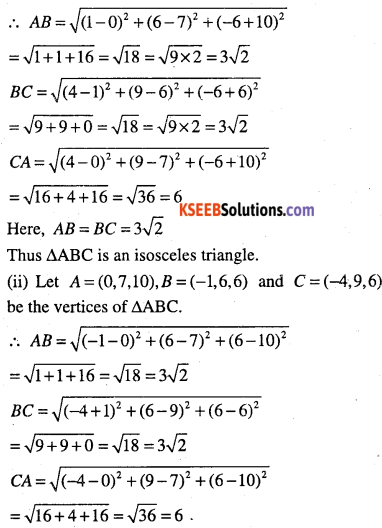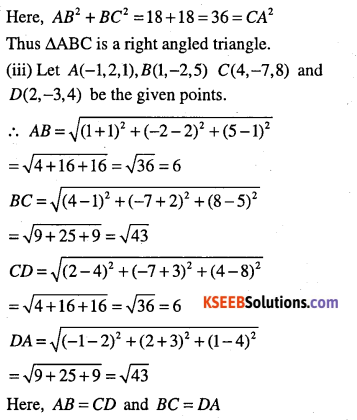i..e., opposite sides of a quadrilateral ABCD are equal, therefore ABCD is a parallelogram.

Question 14.
Are the points A (3, 6, 9), B (10, 20, 30) C (25, -41, 5). The vertices of a right angled triangle.
By distance formula,
AB2 = (10 – 3)2 + (20 – 6)2 + (30 – 9)2
= 49 + 196 + 441 = 686
BC2 = (25 -10)2 + (- 41 – 20)2 + (5 – 30)2
= 225 + 3721 + 625 = 4571
CA2 = (3 – 25)2 + (6 + 41)2 + (9 – 5)2
= 484 + 2209 + 16 = 2709
We find that AB2 + CA2 ≠ BC2
(∵ 3395 ≠ 4571)
∴ ABC is not a right angled triangle.

Question 15.
Find the equation of the set of points which are equidistant from the points (1, 2, 3) and (3,2,-1).
Let A (1, 2, 3) and B (3, 2, -1) be the given points.
Let P(x, y, z) be any point equidistant from A and B, then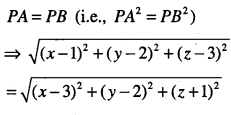⇒ x2-2x + 1+ y2 – 4y + 4 + z2 + 2z + 1
⇒ x2-6x + 9+y2-4y + 4 + z2 + 2z + 1
⇒ 4x – 8z = 0
⇒ x – 2z = 0 is the required equation.Question 16.
Find the equation of the set of points P, the sum of whose distances from A (4, 0, 0) and B (-4,0,0) is equal to 10.
Given points are A (4, 0, 0) and B (-4, 0, 0).
Let P(x, y, z) be any point such that
PA + PB = 10
⇒ PA = 10 – PB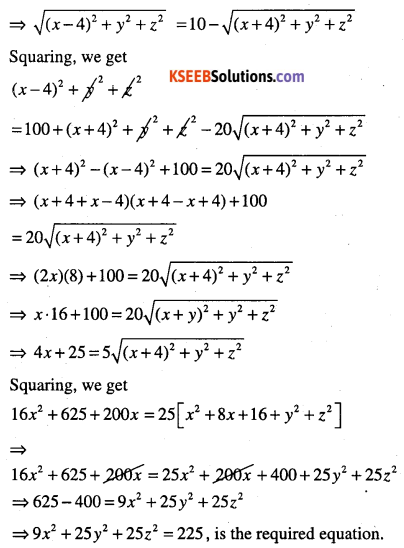Question 17.
Find the equation of set of points P such that PA2 + PB2 = 2k2, where A and B are the points (3,4,5) and (-1,3, -7), respectively.
Let P = (x,y,z), B = (3,4,5) and B = (-1,3-7).
Now, PB2 = (x +1)2 + (y – 3)2 + (z + 7)2 and PA2
= (x – 3)2 + (y – 4)2 + (z – 5)2
Given that PA2 + PB2 = 2k2, then
(x-3)2 + (y-4)2 +(z-5)2 + (x + 1)2 +(y-3)2+(z + 7)2 = 2k2
⇒ x2 – 6*+ 9+ y2 – 8y + 16 + z2 – 10z + 22 + x2 +2x +1 +
y2 – 6y + 9 + z2 +14z + 49 = 2k.
⇒ 2x2 + 2y2 + 2z2 – 4x – 14y + 4z + 109 = 2k2
⇒ 2x2 + 2y2 + 2z2 – Ax – 14y + 4z = 2k2 -109Question 18.
Find the coordinates of the point which divides the line segment joining the points
(-2, 3, 5) and (1, -4, 6) in the ratio
(i) 2 : 3 internally
(ii) 2:3 externally.
The given points are A (-2, 3, 5) and B (1, -4, 6).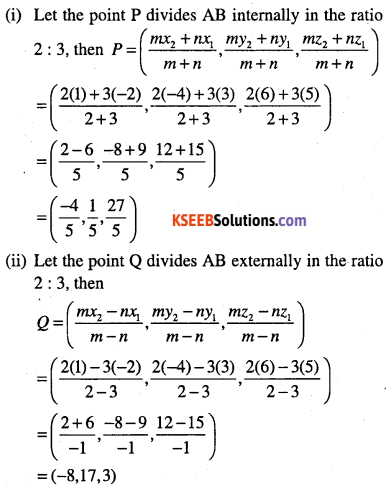Question 19.
Find the coordinates of the point which divides the line segment joining the points
(1, -2, 3) and (3, 4, -5) in the ratio 2 : 3
(i) internally and
(ii) externally.
Try yourself.
$$\text { (i) }\left(\frac{9}{5}, \frac{2}{5}, \frac{-1}{5}\right) \text { (ii) }(-3,-14,19)$$

Question 20.
Given that P (3, 2, -4), Q (5, 4, -6) and R (9, 8, -10) are collinear. Find the ratio in which Q divides PR.
Let Q divides PR in the ratio k : 1, then Q is of the form,
$$Q=\left(\frac{k x_{2}+x_{1}}{k+1}, \frac{k y_{2}+y_{1}}{k+1}, \frac{k z_{2}+z_{1}}{k+1}\right)$$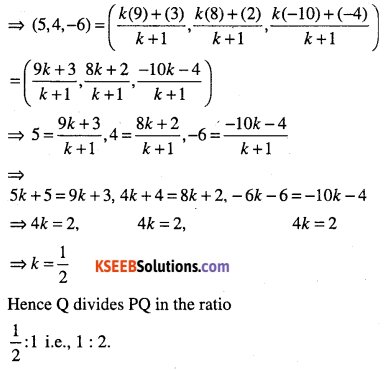Question 21.
Find the ratio in which the yz-plane divides the line segment formed by joining the points (-2,4,7) and (3,-5, 8)
Let yz – plane divides the line segment joining A (-2, 4, 7) and B (3, -5, 8) at P(x,y,z) in the ratio k : 1. Then the coordinates of P are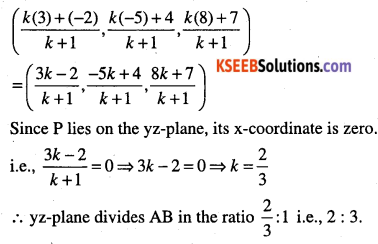Question 22.
Find the ratio in which the line segment joining the points (4, 8, 10) and (6, 10, -8) is divided by the yz-plane.
Let yz – plane divides the line segment joining (4, 8, 10) and (6, 10, -8) at P (x, y, z) in the ratio k : 1, then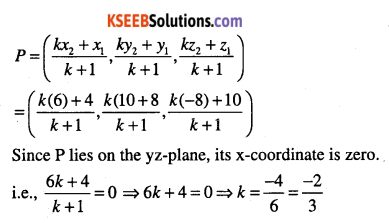∴ yz – plan divided AB externally in the ratio 2 : 3 $$\left(\ k=\frac{-2}{3}<0\right)$$Question 23.
Using section formula, show that the points A(2,-3,4), B(-1,2,1) and $$C\left(0, \frac{1}{3}, 2\right)$$
A(2,-3,4), B(-1,2,1) and $$C\left(0, \frac{1}{3}, 2\right)$$ be the ratio k : 1 ,Then coordinates of the points P are,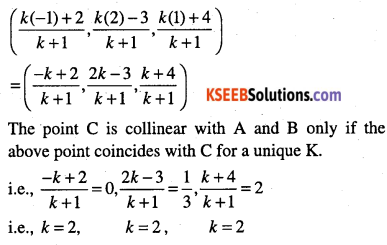Question 24.
Find the coordinates of the points which trisects the line segment joining the points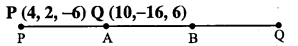Given points P (4, 2, -6) and Q (10, -16, 6).
Let A and B be the points of trisection of PQ, then A divides PQ in the ratio 1 : 2 and B divides PQ in the ratio 2:1.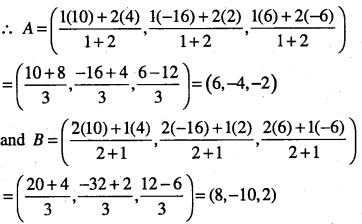Miscellaneous Examples

Question 1.
Show that the points A (1,2, 3), B (-1, -2, -1), C (2, 3, 2) and D (4, 7, 6) are the vertices of a parallelogram ABCD, but it is not a rectangle.
Given points are A (1, 2, 3), B (-1, -2, -1), C (2, 3, 2) and D (4, 7, 6).
To show ABCD is a parallelogram we need to show opposite sides are equal.
By distance formula, we have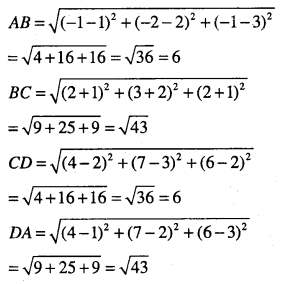Since, AB = CD and BC = DA. ABCD is a parallelogram.
Now, it is required to prove that ABCD is not a rectangle. For this, we show that diagonals AC and BD are unequal.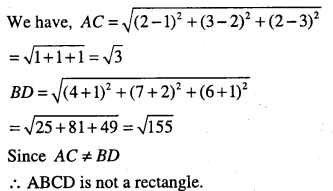Question 2.
Three vertices of a parallelogram ABCD are A (3, -1, 2), B (1, 2, -4) and C (-1, 1, 2). Find the co-ordinates of the fourths vertex.
Let the fourth vertex be D(x1 , y1, z1) we know that diagonals of a parallelogram bisect each other. So the mid-point of the diagonal AC is same as the mid-point of the diagonal BD.
∴ By mid-point formula, we have
$$\left(\frac{3+(-1)}{2}, \frac{-1+1}{2}+\frac{2+2}{2}\right)=\left(\frac{x_{1}+1}{2}, \frac{y_{1}+2}{2}, \frac{z_{1}-4}{2}\right)$$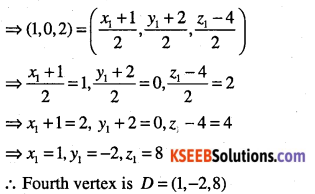Question 3.
Find the lengths of the medians of the triangle with vertices A (0, 0, 6), B (0, 4, 0) and C (6,0,0).
Given (points) vertices A are A (0, 0, 6), B (0, 4, 0) and C (6, 0, 0).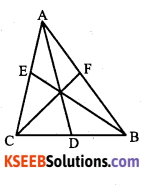Let AD, BE and CF be the medians of the ΔABC. Then, D, E and F are the mid-points of the sides BC, CA and AB respectively.
∴ By mid-point formula, we have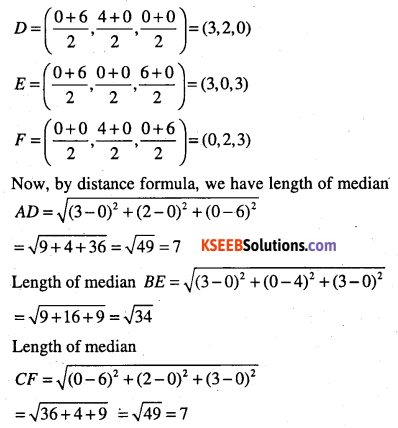Question 4.
If the origin is the centroid of the triangle PQR with vertices P (2a, 2, 6),
Q (-4, 3b, -10)and R (8, 14, 2c), then find the values of a, b and c.
Given vertices are P (2a, 2, 6), Q (-4, 3b, -10) and R (8, 14, 2c).
Let G be the centroid of the ΔPQR then G = (0, 0, 0) (given).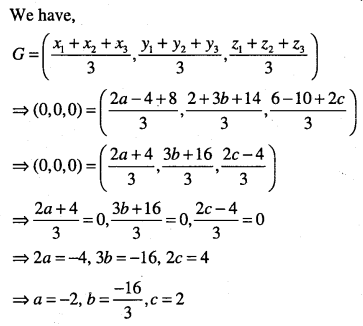Question 5.
The centroid of a triangle ABC is at the point (1, 1, 1). If the coordinates of A and B are (3, -5, 7) and (-1, 7, -6) respectively, find the coordinates of the point C.
Let C = (x,y,z) and centroid G = (1,1,1). Then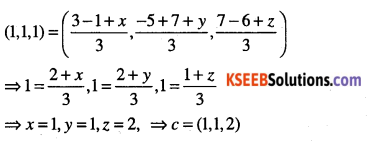Question 6.
Find the coordinates of a point on the y-axis which are at a distance of $$5 \sqrt{2}$$from the point P (3, -2,5).
Given point is P (3, -2, 8)
Let Q be the point on the y-axis then Q be (0, y, 0).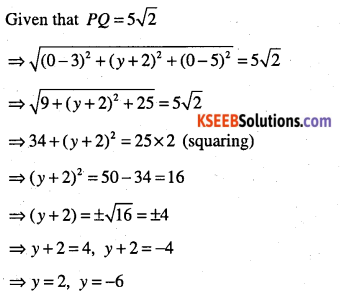∴ Required point on the y – axis is either (0,2,0) or (0,-6, 0)

Question 7.
A point R with x-coordinate 4 lies on the line segment joining the points P (2, -3, 4) and Q (8, 0, 10). Find the coordinates of the point R.
Since R lies on the line PQ, then R divides PQ in some ratio say k : 1.
∴ By section formula R is of the form,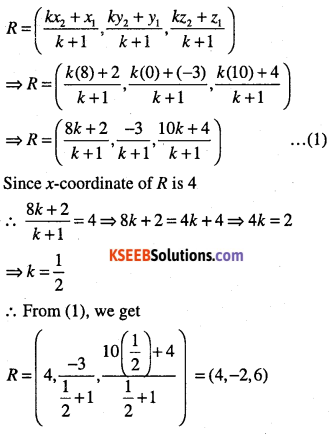Question 8.
If A and B be the points (3, 4, 5) and (-1, 3, -7) respectively, find the equation of the set of points P such that PA2 +PB2 =k2 where k is constant.
Given points are A (3, 4, 5) and B (-1, 3, -7).
Let P = (x,y,z)
Given: PA2 + PB2 = k2
⇒ (x-3)2 + (y-4)2 + (z-5)2 +(x + 1)2 + (y – 3)2 + (z + 7)2 =k2
⇒ 2x1 – 6x + 9 + y2 -8y + 16 + z2 -10z + 25 + x2 + 2x +
1+y2 – 6y + 9 + z2 +14z + 49 = k2
⇒ 2x2 + 2y2 + 2z2 – 4x – 14y + 4z +109 = k2
∴ Required equations of the set of points P is,
2x2 + 2y2 +2z2 – 4x – 14y + 4z + 109 – k2 =0

Question 9.
Find the equation of the set of points P such that PA = PB, where A (3, 4, -5) and
B (-2,1,4).